Band structure and carrier concentration

Basic Parameters
Band structure
Intrinsic carrier concentration
Effective Density of States in the Conduction and Valence Band
Temperature Dependences
Dependences on Hydrostatic Pressure
Energy Gap Narrowing at High Doping Levels
Effective Masses and Density of States
Donors and Acceptors
More than 200 different polytypes of SiC are known. However, about 95% of all publications deal with three main polytypes: 3C, 4H, and 6H. In all main polytypes of SiC, some atoms have been observed in association both with cubic (C), with hexagonal (H) and with rombohedral (R) lattice sites.

Basic Parameters

 3C-SiC: cubic unit cell (Zincblende) Remarks Referens Energy gaps, Egind(Γ15v -X1c) 2.416(1) eV 2 K, wevelength modulated absorption Bimberg et al.(1981) Energy gaps, Eg 2.36 eV 300 K Goldberg et al.(2001) Energy gaps, Egdir(Γ15v -X1c) 6.0 eV 300 K, optical absorption Dalven (1965) Excitonic Energy gaps, Egx 2.38807(3) eV 1.4 K, wevelength modulated absorption see also Temperature Dependences Gorban' et al.(1984)
 Conduction band Remarks Referens Energy separation between  Γ15v valley and L1c valleys EL 4.6 eV 300 K Goldberg et al.(2001) Energy separation between  Γ15v valley and Γ1c valleys EΓ 6.0 eV 300 K Valence band Energy of spin-orbital splitting Eso 0.01 eV 300 K Goldberg et al.(2001) Energy of crystal-field splitting Ecr --- Effective conduction band density of states 1.5 x 1019cm-3 300 K Effective valence band density of states 1.2 x 1019 cm-3 300 K

 2H-SiC: Hexagonal unit cell (Wurtzite) Remarks Referens Excitonic Energy gaps, Eg 3.330 eV optical absorption see also Temperature Dependences Patrick et al.(1966)

 4H-SiC: Hexagonal unit cell (Wurtzite) Remarks Referens Energy gaps, Eg 3.23 eV 300 K Goldberg et al.(2001) Excitonic Energy gaps, Egx 3.20 eV see also Temperature Dependences Dubrovskii & Lepneva (1977)
 Conduction band Remarks Referens Energy separation between  Γ15v valley and L valleys EL ~=4. eV 300 K Goldberg et al.(2001) Energy separation between  Γ15v valley and Γ1c valleys EΓ 5-6.0 eV 300 K Valence band Energy of spin-orbital splitting Eso 0.007 eV 300 K Goldberg et al.(2001) Energy of crystal-field splitting Ecr 0.008 eV Effective conduction band density of states 1.7 x 1019cm-3 300 K Effective valence band density of states 2.5 x 1019 cm-3 300 K

 6H-SiC: Hexagonal unit cell (Wurtzite) Remarks Referens Energy gaps, Eg 3.0 eV 300 K Goldberg et al.(2001) Energy gaps, Egind 2.86 eV 300 K, optical absorption see also Temperature Dependences Philipp & Taft (1960) Excitonic Energy gaps, Eg 3.0230 eV wevelength modulated absorption see also Temperature Dependences Humphreys et al.(1981)
 Conduction band Remarks Referens Energy separation between  Γ15v valley and Γ1c valleys EΓ 5-6.0 eV 300 K Goldberg et al.(2001) Valence band Energy of spin-orbital splitting Eso 0.007 eV 300 K Goldberg et al.(2001) Energy of crystal-field splitting Ecr 0.05 eV Effective conduction band density of states 8.9 x 1019cm-3 300 K Effective valence band density of states 2.5 x 1019 cm-3 300 K

 8H-SiC: Hexagonal unit cell (Wurtzite) Remarks Referens Excitonic Energy gaps, Eg 2.86 eV see also Temperature Dependences Dubrovskii & Lepneva (1977)

 15R-SiC: Rhombohedral unit cell Remarks Referens Excitonic Energy gaps, Eg 2.9863 eV wevelength modulated absorption see also Temperature Dependences Humphreys et al.(1981)

 21R-SiC: Rhombohedral unit cell Remarks Referens Excitonic Energy gaps, Eg 2.92 eV see also Temperature Dependences Dubrovskii & Lepneva (1977)

 24R-SiC: Rhombohedral unit cell Remarks Referens Excitonic Energy gaps, Eg 2.80 eV see also Temperature Dependences Dubrovskii & Lepneva (1977)

Band structure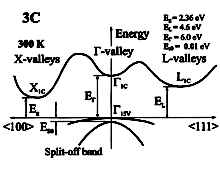SiC, 3C. Band structure. Important minima of the conduction band and maxima of the valence band. .   300K;     Eg = 2.36 eV;       EΓ = 6.0 eV;     EL = 4.6 eV;        Eso = 0.01 eV.  For details see Persson & Lindefelt (1997)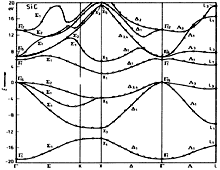SiC, 3C. Band structure Hemstreet & Fong (1974)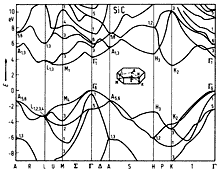SiC, 2H. Band structure Hemstreet & Fong (1974)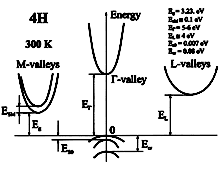SiC, 4H. Band structure. Important minima of the conduction band and maxima of the valence band. .   300K;     Eg = 3.23 eV;       EΓ = 5-6.0 eV;     EL ~= 4.0 eV;        EsM ~= 0.1 eV.      Ecr = 0.08 eV;        Eso = 0.007 eV.  For details see Persson & Lindefelt (1997)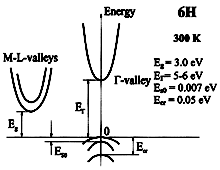SiC, 4H. Band structure. Important minima of the conduction band and maxima of the valence band. .   300K;     Eg = 3.0 eV;       EΓ = 5 - 6.0 eV;     Ecr = 0.05 eV;        Eso = 0.007 eV.  For details see Persson & Lindefelt (1997)

In all polytypcs except 3C- and riH-Sif atomic hiyers wilh cubic (C) and hexagonal (H) symmetry follow in a regular alternation in the direct ion of the c axis. This can be thought of as anutural one-dimensional superkmice imposed on the "pure" i.e. h-layer free 3C-SiC [Dean et al. (1977)], the period of the superlattice being different for different modifications.Brillouin zone of the cubic lattice.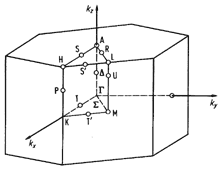Brillouin zone of the hexagonal lattice.

Temperature Dependences

Temperature dependence of energy gap:
 3C-SiC Eg = Eg(0) - 6.0 x 10-4 x T2/(T + 1200) (eV) Goldberg et al.(2001) Egx = 3.024-0.3055x10-4 +T2/(311K - T) (eV) Ravindra & Srivastava (1979) 4H-SiC Eg = Eg(0) - 6.5 x 10-4 x T2/(T + 1300) Goldberg et al.(2001) 6H-SiC Eg = Eg(0) - 6.5 x 10-4 x T2/(T + 1200)
where T is temperature in degrees K.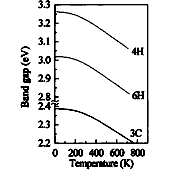SiC, 3C, 4H, 6H. Energy gap vs. temperature. Choyke(1969)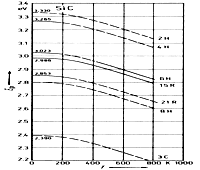SiC, 3C, 15R, 21R, 2H, 4H, 6H, 8H. Excitonic energy gap vs. temperature Choyke(1969)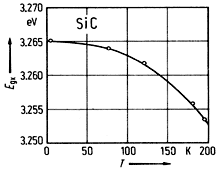SiC, 4H. Excitonic energy gap vs. temperature Choyke et al.(1964)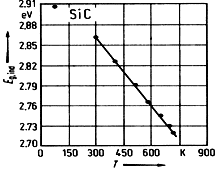SiC, 6H. Energy gap Egind vs. temperature Philipp & Taft(1960)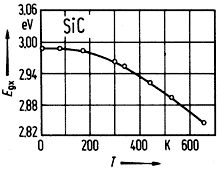SiC, 15R. Excitonic energy gap vs. temperature Patric et al. (1963)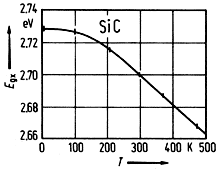SiC, 24R. Excitonic energy gap vs. temperature Zanmarchi (1964)

Intrinsic carrier concentration:

ni = (Nc·Nv)1/2exp(-Eg/(2kBT))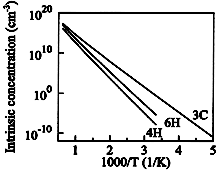SiC, 3C, 4H, 6H. Intrinsic carrier concentration vs. temperature Goldberg et al.(2001)

Effective density of states in the conduction band Nc

3C-SiC

Nc ~= 4.82 x 1015 · M · (mc/m0)3/2·T3/2 (cm-3) ~= 4.82 x 1015 (mcd/m0)3/2·x T3/2 ~= 3 x 1015 x T3/2(cm-3) ,
where M=3 is the number of equivalent valleys in the conduction band.
mc = 0.35m0 is the effective mass of the density of states in one valley of conduction band.
mcd = 0.72 is the effective mass of density of states.

4H-SiC
Nc ~= 4.82 x 1015 · M · (mc/m0)3/2·T3/2 (cm-3) ~= 4.82 x 1015 (mcd/m0)3/2·x T3/2 ~= 3.25 x 1015 x T3/2(cm-3) ,
where M=3 is the number of equivalent valleys in the conduction band.
mc = 0.37m0 is the effective mass of the density of states in one valley of conduction band.
mcd = 0.77 is the effective mass of density of states.
6H-SiC

Nc ~= 4.82 x 1015 · M · (mc/m0)3/2·T3/2 (cm-3) ~= 4.82 x 1015 (mcd/m0)3/2·x T3/2 ~= 1.73 x 1015 x T3/2(cm-3) ,
where M=6 is the number of equivalent valleys in the conduction band.
mc = 0.71m0 is the effective mass of the density of states in one valley of conduction band.
mcd = 2.34 is the effective mass of density of states.

Effective density of states in the valence band Nv

 3C-SiC Nv = 2.23x1015 x T3/2 (cm-3) Ruff et al. (1994), Casady and Johnson (1996) 4H-SiC Nv ~= 4.8x1015 x T3/2 (cm-3) 6H-SiC Nv ~= 4.8x1015 x T3/2 (cm-3)

Dependence on Hydrostatic Pressure

 3C-SiC Eg = Eg(0) - 0.34 x 10-3P (eV) Park et al. (1994) EL = EL(0) + 3.92 x 10-3P (eV) EΓ = EΓ(0) + 5.11 x 10-3P (eV) 4H-SiC Eg = Eg(0) + 0.8 x 10-3P (eV) EΓ = EΓ(0) + 3.7 x 10-3P (eV) 6H-SiC Eg = Eg(0) - 0.03 x 10-3P (eV) EΓ = EΓ(0) + 4.03 x 10-3P (eV)
here P is pressure in kbar Park et al. (1994).

Energy Gap Narrowing at High Doping Levels: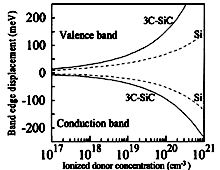3C-SiC. Conduction and valence band displacements vs. ionized shallow impurity. n-type material. For comparison, the band-edge displacements for Si are shown Lindefelt (1998)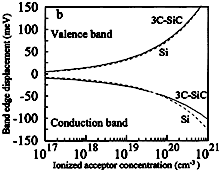3C-SiC. Conduction and valence band displacements vs. ionized shallow impurity. p-type material. For comparison, the band-edge displacements for Si are shown Lindefelt (1998)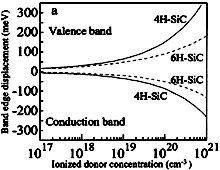4H-SiC, 6H-SiC. Conduction and valence band displacements vs. ionized shallow impurity. n-type material. For comparison, the band-edge displacements for Si are shown Lindefelt (1998)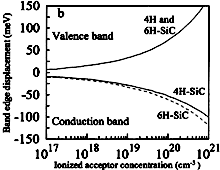4H-SiC, 6H-SiC. Conduction and valence band displacements vs. ionized shallow impurity. p-type material. For comparison, the band-edge displacements for Si are shown Lindefelt (1998)

The band-edge displacements for n-type material Lindefelt (1998) :
ΔEnc=Anc(ND+)1/3 x 10-6 + Bnc(ND+)1/2 x 10-9 (eV)
ΔEnv=Anv(ND+)1/3 x 10-6 + Bnv(ND+)1/2 x 10-9 (eV)
where

 n-type Anc Bnc Anv Bnv Si -9.74x10-3 -1.39x10-3 -1.27x10-2 -1.40x10-3 3C-SiC -1.48x10-2 -3.06x10-3 -1.75x10-2 -6.85x10-3 4C-SiC -1.50x10-2 -2.93x10-3 -1.90x10-2 -8.74x10-3 6C-SiC -1.12x10-2 -1.01x10-3 -2.11x10-2 -1.73x10-3

The band-edge displacements for p-type material Lindefelt (1998) :
ΔEpc=Apc(ND+)1/3 x 10-6 + Bpc(ND+)1/2 x 10-9 (eV)
ΔEpv=Apv(ND+)1/3 x 10-6 + Bpv(ND+)1/2 x 10-9 (eV)
where

 p-type Apc Bpc Apv Bpv Si -1.14x10-3 -2.05x10-3 -1.11x10-2 -2.06x10-3 3C-SiC -1.50x10-2 -6.41x10-4 -1.30x10-2 -1.43x10-3 4C-SiC -1.57x10-2 -3.87x10-4 -1.30x10-2 -1.15x10-3 6C-SiC -1.74x10-2 -6.64x10-4 -1.30x10-2 -1.14x10-3

Effective Masses and Density of States:

Electrons

3C-SiC. The surfaces of equal energy are ellipsoids:
 3C-SiC Remarks Referens Effective electron mass       (longitudinal)ml 0.68mo Son et al. (1994); Son et al. (1995) 0.677(15)mo 45K, Cyclotrone resonance Kaplan et al. (1985) Effective electron mass       (transverse)mt 0.25mo Son et al. (1994); Son et al. (1995) 0.247(11)mo 45K, Cyclotrone resonance Kaplan et al. (1985) Effective mass of density of states  mcd 0.72mo Son et al. (1994); Son et al. (1995) Effective mass of the density of states in one valley of conduction band mc 0.35mo Effective mass of conductivity  mcc 0.32mo
There are 3 equivalent valleys in conduction band

4H-SiC. The surfaces of equal energy are ellipsoids:
 4H-SiC Remarks Referens Effective electron mass       (longitudinal)ml 0.29mo Son et al. (1994); Son et al. (1995) Effective electron mass       (transverse)mt 0.42mo Son et al. (1994); Son et al. (1995) Effective mass of density of states  mcd 0.77mo Son et al. (1994); Son et al. (1995) Effective mass of the density of states in one valley of conduction band mc 0.37mo Effective mass of conductivity  mcc 0.36mo
There are 3 equivalent valleys in conduction band

6H-SiC. The surfaces of equal energy are ellipsoids:
 6H-SiC Remarks Referens Effective electron mass       (longitudinal)ml 0.20mo Son et al. (1994); Son et al. (1995) Effective electron mass       (transverse)mt 0.42mo Son et al. (1994); Son et al. (1995) Effective mass of density of states  mcd 2.34mo Son et al. (1994); Son et al. (1995) Effective mass of the density of states in one valley of conduction band mc 0.71mo Effective mass of conductivity  mcc 0.57mo
There are 6 equivalent valleys in conduction band

Holes

Due to the small spin-orbit splitting the valence bands are highly non-parabolic, i.e. effective hole masses become strongly k-dependent.
 SiC Remarks Referens 3C Effective mass of density of state mvĀ 0.6 mo 300 K Son et al. (1994); Son et al. (1995) 4H Effective mass of density of state mvĀ ~1.0 mo 300 K 6H Effective mass of density of state mv ~1.0 mo 300 K